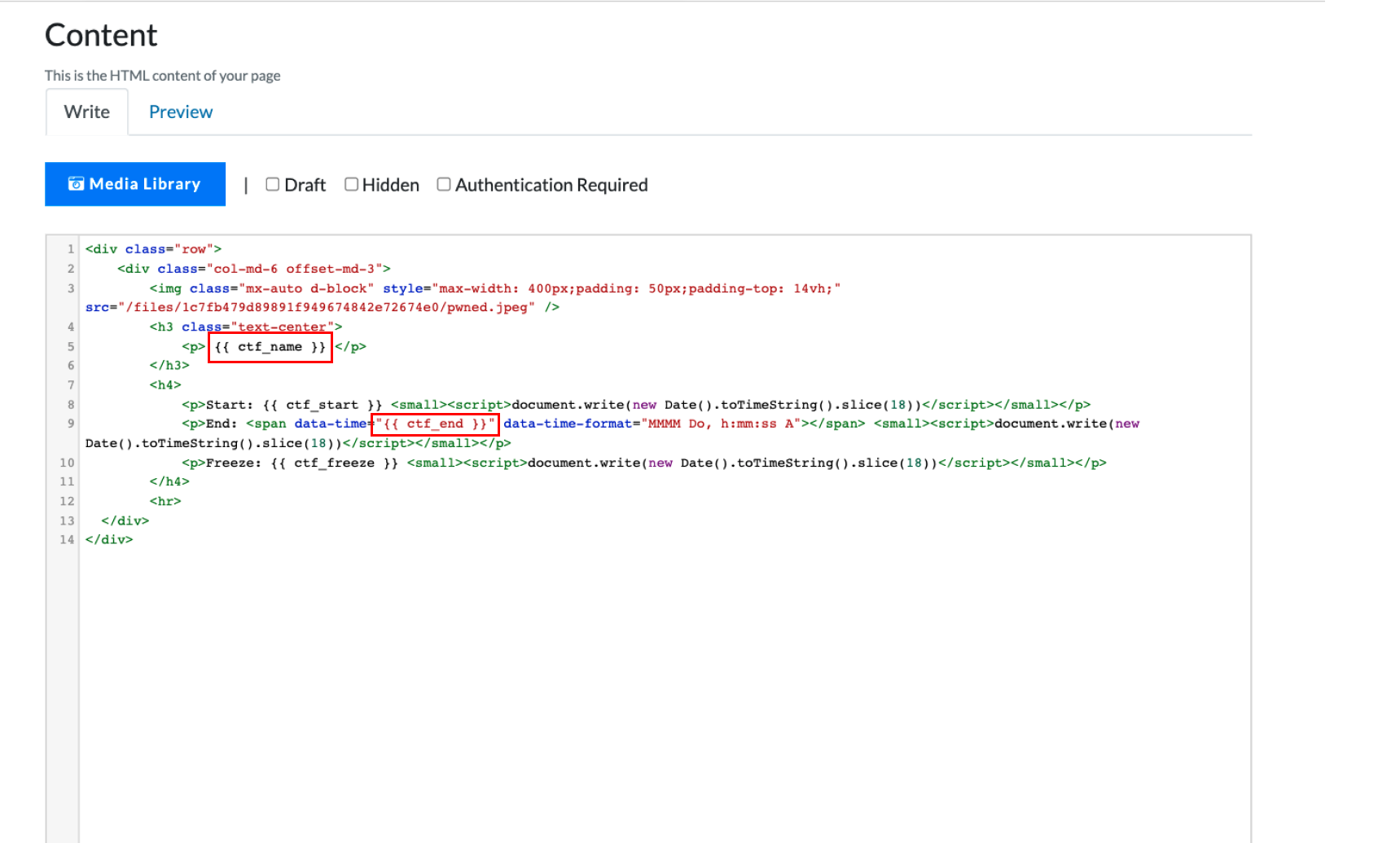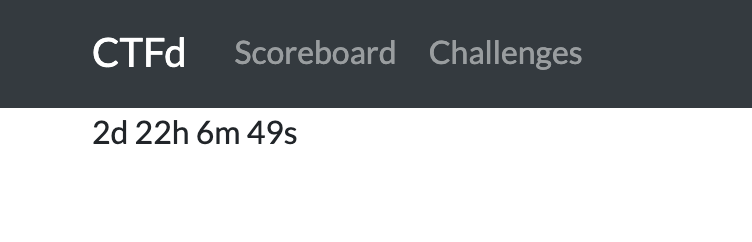# Page Variables

Pages have access to variables that are inserted into content dynamically. These variables are configured through the CTFd configuration page and then inserted into the HTML when it's loaded.

Variables can be accessed as `{{ variable }}`.

For example if your variable is `ctf_name`, it can be inserted into Page content as `{{ ctf_name }}` as shown below.### Available Variables​

`ctf_name` - The event name

`ctf_description` - The event description

`ctf_start` - The event start time as an ISO8601 timestamp (e.g. `2021-12-21T01:40:00Z`)

`ctf_end` - The event end time as an ISO8601 timestamp (e.g. `2021-12-21T01:40:00Z`)

`ctf_freeze` - The event freeze time as an ISO8601 timestamp (e.g. `2021-12-21T01:40:00Z`)

### Examples​

#### Countdown Timer​

Install a countdown timer on any Page by copying the below code into any Page and configuring the CTF Start Time in the Config Panel.``<!-- https://www.w3schools.com/howto/howto_js_countdown.asp --><p id="demo"></p><script>  // Set the date we're counting down to  var countDownDate = new Date("{{ ctf_start }}").getTime();  // Update the count down every 1 second  var x = setInterval(function () {    // Get today's date and time    var now = new Date().getTime();    // Find the distance between now and the count down date    var distance = countDownDate - now;    // Time calculations for days, hours, minutes and seconds    var days = Math.floor(distance / (1000 * 60 * 60 * 24));    var hours = Math.floor(      (distance % (1000 * 60 * 60 * 24)) / (1000 * 60 * 60)    );    var minutes = Math.floor((distance % (1000 * 60 * 60)) / (1000 * 60));    var seconds = Math.floor((distance % (1000 * 60)) / 1000);    // Display the result in the element with id="demo"    document.getElementById("demo").innerHTML =      days + "d " + hours + "h " + minutes + "m " + seconds + "s ";    // If the count down is finished, write some text    if (distance < 0) {      clearInterval(x);      document.getElementById("demo").innerHTML = "EXPIRED";    }  }, 1000);</script>``